# a student project course page 2 of 3

Status
Not open for further replies.

#### hobbyist

Joined Aug 10, 2008
892
lets take a look: pic.9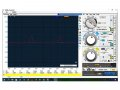much better, no clipping and were still holding about 6v. p-p.
I'll stay with those values.
that finishes this building block.

So here we show our pulse generatore output, and the RC network output on the scope. pic.10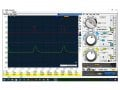We now have a 2mS pulse with a 20mS period, feeding into a RC network producing a ramp pulse with a pointy top and a base width of around 4mS which will be plenty good to drive the next stage.

This next building block is a Digital circuit, it takes a analog signal, in this case a ramp sawtooth wave, and converts it into a digital high low signal, either a one or a zero on its output. by the time we get this building block built, we will be able to have a PWM output however it will still need some conditioning to be usefull to drive a micro circuit in a servo motor.

Here is the next stage of design:

Analog to digital circuit.

we will now design a schmitt trigger circuit, however since I am not going to go into all the detailed equations given in my course design books from (NRI) course. , I'll use a short version of my own equations to get reasonable results, which is good enough for this application.
The design is not my own, just the formulas used to find the resistor values.
Here are the equations I came up with to build this circuit.

refer to circuit below.

1.) VCC is chosen value.
2.) Vth = (Vcc / 2) (threshold, trigger voltage)
3.) R7 is chosen value.
vbe = 0.7v. and vce = 0.3v. (respectively base emitter voltage and collector emitter saturation voltage)
4.) VR8 = (Vth - vbe)
5.) R8 = {(VR8 * R7) / (VCC - (VR8 +vce)}
6.) R9 = (10 * R8)
7.) IR9 = (Vth / R9)
8.) R10 = (0.1v / IR9)
9.) R11 = {(VCC - 0.1v-Vth) / IR9}
so lets now plug in our values to see what resistor values will work for this.
VCC = 8v. (This will allow this circuit to work with a 9 volt battery.)
Vth = 4v.
R7 =470 ohms
R8 = {(3.3v * 470) / (8v - (3.3v +0.3v)} ~=352 ohms, I chose 240 ohm resistor..
R9 = (10* R8) = 2.4K ohms.
IR9 = (4 / 2.4K ohms) ~= 1.7mA.
R10 = (0.1v / 1.7mA) = ~=60 ohms. I chose 62 ohm resistor.
R11 = {(8v-0.1v-4v) / 1.7mA} = ~=2294 ohms I chose a 2.2K ohm resistor. pic.11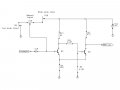The trim resisdtors in series with the manual input pot. is inserted for later tweaking of the circuit for proper input performance.

Ok so now that we have this building block done, lets hook up the preceeding stage to it and see if we get any PWM signal out. pic.12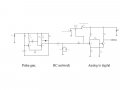When I made this connection as shown above, I ran into a major problem: pic.13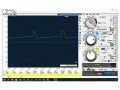Hmm what happened. Well this is one of the problems an electronics engineer will need to deal with on a continuous basis. When designing circuits, you need to keep on thinking about impedance matching, that has several meanings depending on the application.
In rf work it means matching exact values of Zin to Zout. however in analog and digital low frequency work it mainly works out as making sure the input impedance your working into is always higher then the signal source working into it.

The signal source in this case is the RC integrator stage, it has an output impedance (Zout) of around 3K ohms. (resistor R5)
The input impedance (Zin) of the analog to digital convertor block (ADC) has an input impedance that varies from (0v. to around (the parallel combination of 11K pot value and the 240 ohm emitter resistor in the be junction of Q3.))

In other words we need some sort of a buffer between these two stages, between the RC network and the ADC stage.
There are several options, using mosfet is one of them, however trying to keep this at a bipolar transistor level only, lets use a emitter follower (CC) building block.

A rule of thumb for matching impedances at low frequencies, is to make the input impedance of the stage the signal source is feeding into to be 10 times or higher than the output impedance of the signal source, thats feeding it.

looking back we see the 3K ohm resitor (R5) as the Zout of the RC stage, so lets make Zin of the (CC) stage to be around 30K or more.
So if we use two 62K ohm resitors to form a voltage divider across the base of the transistor (CC) than the parallel combination gives around 30K ohms.
Assuming a dc beta of 100 for a transistor (2n3904) for calculating purposes, then we can use a resitor in its emitter of around 300 ohms to make the input look like a 30K ohm resistor, or somewhere in that range.

Now if we use an emitter resitor of a value 1/10th of the pull up resitor (R13) (62K), that would be a value of (6.2K ohms).
So we are keeping our Z matching consistent somewhat, where the emitter resistor is ten times less than the pull up resistor, and the parallel combo. of the volt. divider is around ten times larger than the 3K ohm resistor of the RC network, we should have good signal flow with little distortion.
lets build this (CC) buffer building block to see how this works out. pic.14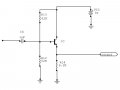pic.15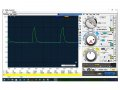Ok we have our waveform back in working order, its peak voltage is around 6-7v. with nice sharp point on top.
Now lets see how we do on the (ADC) building block.

When I hook it up, the (ADC) goes full positive voltage, so there is no variable pulse width, yet. In other words it is overdriving the next stage.
OK so now we use some logic thinking, since my (ADC) is designed to trigger at 4v, and my triangle wave peaks at around 6-7v., then I need to make the pk value of this wave much less than 4v. in order to not overdrive the (ADC) stage.

That's where you use attenuation networks. A simple voltage divider is used to divide the output voltage of the RC network down to a value much lesser than 4v.

Lets choose the triangle wave pk. volt will be attenuated down to around 2volts, or so.
Lets insert this attenuator between the (RC) stage and the (CC) stage.

These values are chosen like this, I make R15 to be 30K ohms so it is ten times larger than R5 than I empirically insert a ground resistor and watch my waveform, when it is peaking around 2v. than that is the resistor combination I use to do the attenuation with.
In my case I found that a 30K ohm resitor to ground gave me close to 2v. pk. on the triangle wave. C4 is inserted to block any dc bias of the (CC) stage from interfering with the (RC) stage, it is known as a coupling capacitor, it couples the ac (triangle wave) to the next stage while blocking dc voltages.
So this is what the attenuation network looks like between the 2 stages.

The atten. are comprised of (R15 and R16): pic.16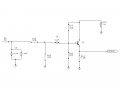Now lets see where, were at on the waveforms.
First we need to tweak those trim pots. I was talking about earlier.
NOTE:
Also not shown you may need to use larger value trim pots. I had to change mine to larger values, I just put those values shown on the circuit schematic as a ballpark figure. If you don't have larger trim pots. than add series resistors to the trim pots.

to be continued on page 3 of 3 stay tuned

Status
Not open for further replies.